# Refraction of Light

### Guest Account

Attempt Form Three Physics Questions
Guest Account

# Refraction of Light

### Introduction

Refraction is the change of direction of light rays as they pass at an angle from one medium to another of different optical densities

Explanation: To investigate the path of light through rectangular glass block.
Apparatus: - soft-board, white sheet of paper, drawing pins (optical), rectangular glass block.
Procedure
1. Fix the white plain paper on the soft board using pins
2. Place the glass block on the paper and trace its outline, label it ABCD as shown below
3. Draw a normal NON at point O
4. Replace the glass block to its original position
5. Stick two pins P1 and P2 on the line such that they are at least 6cm apart and upright
6. Viewing pins P1 and P2 from opposite side, fix pins P3 and P4 such that they’re in a straight line
7. Remove the pins and the glass block
8. Draw a line joining P3 and P4 and produce it to meet the outline face AB at point O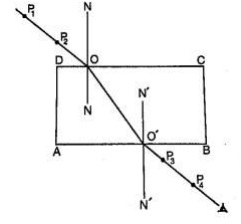Explanation of Refraction
- Light travels at a velocity of 3.0 & 108 in a vacuum.
- Light travels with different velocities in different media.
- When a ray of light travels from an optically less dense media to more dense media, it is refracted towards the normal.
- The glass block experiment gives rise to a very important law known as the law of reversibility which states that "if a ray of light is reversed, it always travels along its original path".
- If the glass block is parallel-sided, the emergent ray will be parallel to the incident ray but displaced laterally as shown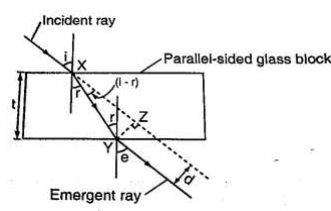'e' is called the angle of emergence
The direction of the light is not altered but displaced sideways.
This displacement is called lateral displacement and is denoted by 'd'. Therefore
XY = t/Cos r, YZ = Sin (i - r) × x y
So, lateral displacement, d = t Sin (i - r)/Cos r

### Laws of Refraction

1. The incident ray, the refracted ray and the normal at the point of incidence all lie on the same plane.
2. The ratio of the sine of the angle of incidence to the sine of the angle of refraction is a constant for a given pair of media.
Sin i/sin r = constant (k)

### Refractive index

Refractive index (n) is the constant of proportionality in Snell's law: hence

Sin i/ sin r = n
Therefore sin i/sin r = n = 1/sin r/sin i

Example 1
Calculate the refractive index for light travelling from glass to air given that ang = 1.5
Solution

gna= 1/ang = 1/1.5 = 0.67

Example 2
Calculate the angle of refraction for a ray of light from air striking an air-glass interface, making an angle of 600 with the interface. (ang = 1.5
Solution

Angle of incidence (i) = 900 - 600 = 300
1.5 = sin 30°/sin r, sin r = sin 300/1.5 = 0.5/1.5
Sin r = 0.3333, sin-10.3333 = 19.50
R = 19.50

### Refractive Index In Terms Of Velocity

Refractive index can be given in terms of velocity by the use of the following equation;
n2 = velocity of light in medium 1/velocity of light in medium 2
When a ray of light is travelling from vacuum to a medium the refractive index is referred to as absolute refractive index of the medium denoted by 'n'
Refractive index of a material 'n' = velocity of light in a vacuum/velocity of light in material 'n'
The absolute refractive indices of some common materials is given
Material Refractive Index
Air (ATP) 1.00028
Ice 1.31
Water 1.33
Ethanol 1.36
Kerosene 1.44
Glycerol 1.47
Perspex 1.49
Glass (crown) 1.55
Glass (flint) 1.65
Ruby 1.76
Diamond 2.72

Example 3
A ray of light is incident on a water-glass interface as shown. Calculate 'r'. (Take the refractive index of glass and water as 3/2 and 4/3 respectively)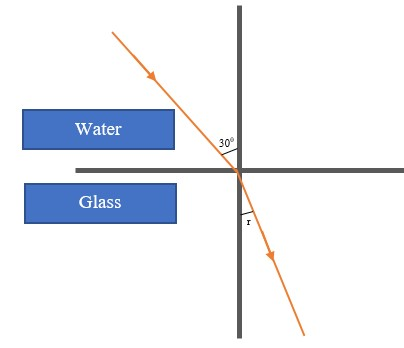Solution

Since anw sin θw = ang sing
4/3 sin 300 = 3/2 sin r
3/2 sin r = 4/3 × 0.5
Sin r = 4/6 × 2/3 = 4/9 = 0.4444
r = 26.40

Example 4
The refractive index of water is 4/3 and that of glass is 3/2. Calculate the refractive index of glass with respect to water.
Solution

wng = gna × ang, but wna = 1/anw = 3/4
wng = 3/4 × 3/2 = 9/8 = 1.13

Real and apparent depth
Consider the following diagram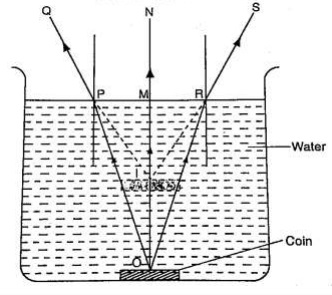- The depth of the water OM is the real depth, and the distance IM is known as the apparent depth.
- OI is the distance through which the coin has been displaced and is known as the vertical displacement.
- The relationship between refractive index and the apparent depth is given by;
Refractive index of a material = real depth/apparent depth
Example 5
A glass block of thickness 12 cm is placed on a mark drawn on a plain paper. The mark is viewed normally through the glass. Calculate the apparent depth of the mark and hence the vertical displacement. (Refractive index of glass =3/2)
Solution

ang = real depth/apparent depth
apparent depth = real depth/ang = (12 × 2)/3 = 8 cm
vertical displacement= 12 - 8 = 4 cm

Applications Of Refractive Index
Total internal reflection

- This occurs when light travels from a denser optical medium to a less dense medium.
- The refracted ray moves away from the normal until a critical angle is reached usually 900 where the refracted ray is parallel to the boundary between the two media.
- If this critical angle is exceeded total internal reflection occurs and at this point no refraction occurs but the ray is reflected internally within the denser medium.

### Relationship Between The Critical Angle And Refractive Index

Consider the following diagram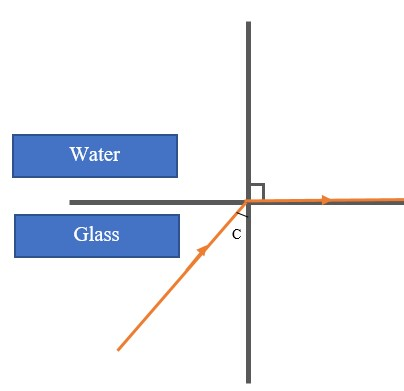From Snell’s law
gnw = sin C/sin 900,but ang = 1/gna since sin 900 = 1
Therefore ang= 1/sin C, hence sin C=1/n or n=1/sin C

Example 6
Calculate the critical angle of diamond given that its refractive index is 2.42
Solution

Sin C = 1/n = 1/ 2.42 = 0.4132 = 24.40

Effects Of Total Internal Reflection
1. Mirage: These are ‘pools of water’ seen on a tarmac road during a hot day. They are also observed in very cold regions but the light curves in opposite direction such that a polar bear seems to be upside down in the sky.
2. Atmospheric refraction: the earths’ atmosphere refracts light rays so that the sun can be seen even when it has set. Similarly the sun is seen before it actually rises.

#### Applications Of Total Internal Reflection

1. Periscope: a prism periscope consists of two right angled glass prisms of angles 450,900 and 450 arranged as shown below.
They are used to observe distant objects.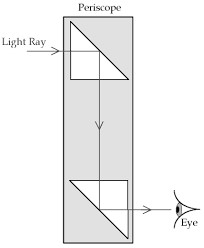2. Prism binoculars: the arrangement of lenses and prisms is as shown below. Binoculars reduce the distance of objects such that they seem to be nearer.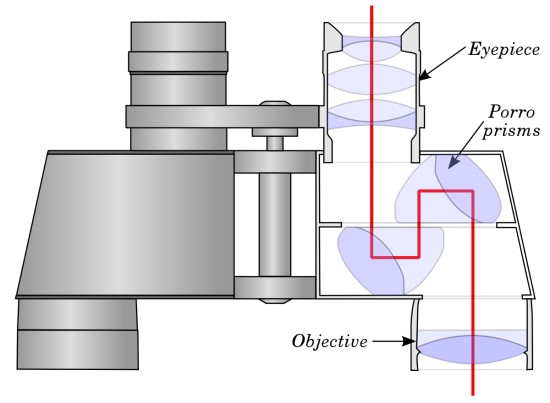Image Courtesy

3. Pentaprism: used in cameras to change the inverted images formed into erect and actual image in front of the photographer.

4. Optical fibre: this is a flexible glass rod of small diameter. A light entering through them undergoes repeated internal reflections. They are used in medicine to observe or view.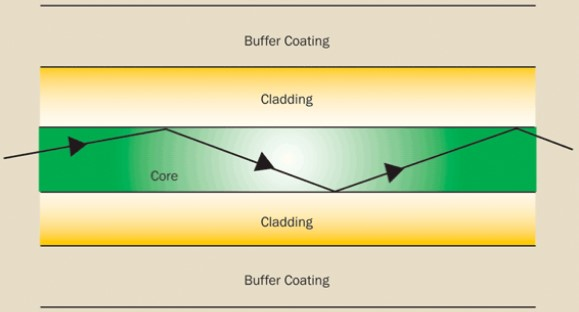Image Courtesy

5. Dispersion of white light: the splitting of light into its constituent colours is known as dispersion. Each colour represents a different wavelength as they strike the prism and therefore refracted differently as shown.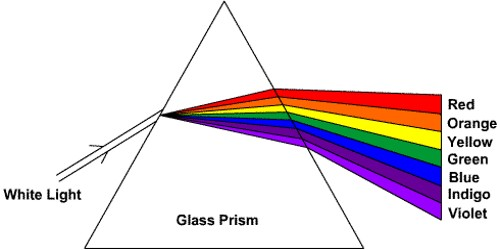Image Courtesy